# KSEEB Solutions for Class 7 Maths Chapter 3 Data Handling Ex 3.3

Students can Download Chapter 3 Data Handling Ex 3.3, Question and Answers, Notes Pdf, KSEEB Solutions for Class 7 Maths, Karnataka State Board Solutions help you to revise complete Syllabus and score more marks in your examinations.

## Karnataka State Syllabus Class 7 Maths Chapter 3 Data Handling Ex 3.3

Question 1.
Use the bar graph (Fig 3.3) to answer the following questions.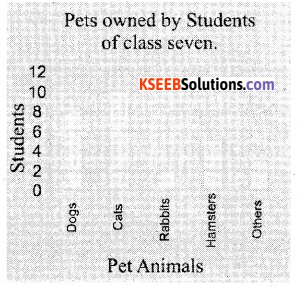i) Which is the most popular pet ?
Solution:
Cat is the most popular pet.

ii) How many students have dog as a pet ?
Solution:
Eight(8) students have dog as a pet.

Question 2.
Read the bar graph (Fig 3.4) which shows the number of books sold by a bookstore during five consecutive years and and answer the following questions: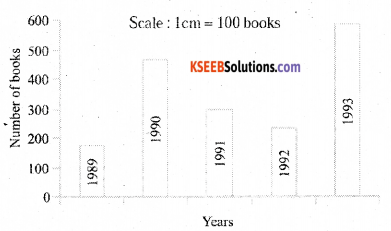i) About how many books were sold in 1989 ?1990 ? 1992 ?
Solution:
Number of books sold in 1989 =180 books approximately.
Number of books sold in 1990 = 475 books approximately.
Number of books sold in 1992 = 225 books approximately.

ii) In which year were about 475 books sold? About 225 books sold ?
Solution:
Approximately 475 books sold in 1990.
Approximately 225 books sold in 1992.

iii) In which years were fewer than 250 books sold ?
Solution:
Less than 250 books sold in the year = 1989 and 1992.

iv) Can you explain how would estimate the number of books sold in 1989 ?
Solution:
The length of bar graph of 1989 is about 1.8cm.
The scale 1cm = 100 books. So number of books = 1.8 × 100 = 180 booksQuestion 3.
Number of children in six different classes are given below. Represent the data on a bar graph.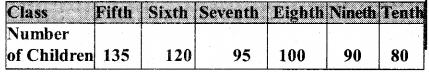Solution:
a) How would you choose a scale ?
Solution:
We should start the scale to represent bar graph is 0. The greatest number is 135. So we choose 1 unit is 10 children. We use equal divisions a? increment of 10. All the bars would be in between 0 and 140.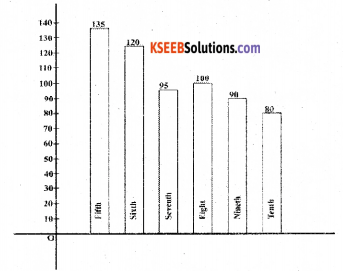i) Which class has the maximum number of children ? And the minimum ?
Solution:
Fifth class has maximum number of chil-dren ie., 135 and Tenth class has minimum number of children ie., 80.ii) Find the ratio of students of class sixth to the students of class eighth.
Solution:Question 4.
The performance of a student in 1st Term and 2nd Term is given. Draw a double bar graph choosing appropriate scale and answer the following :i) In which subject, has the child improved his performance the most ?
Solution:
The child improved his performance the most in mathematics.ii) In which subject is the improvement the least ?
Solution:
The improvement is the least in social science.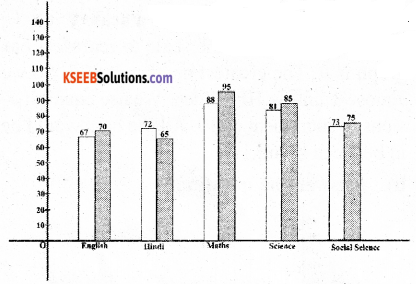iii) Has the performance gone down in any subject ?
Solution:
Yes, the performance has gone down in Hindi.

Question 5.
Consider this data collected from a survey of a colony.
Solution: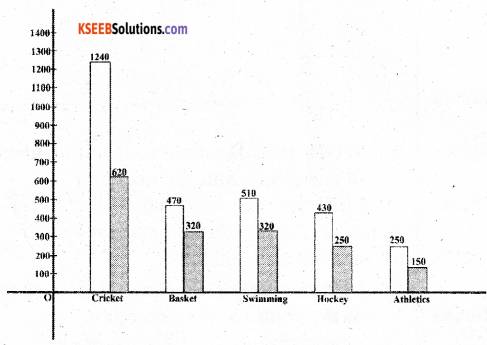i) Draw a double bar graph choosing an appropriate scale.
What do you infer from the bar graph?
Solution:
From the Bar Graph we infer that the viewers for cricket is most and athletics is least.

ii) Which sport is most popular ?
solution:
Cricket is most popular.iii) Which is more preferred, watch or participating in sports ?
Solution:
Watching the sport is more preferred than participating.

Question 6.
Take the data giving the minimum and the maximum temperature of various cities given in the beginning of this Chapter (Table 3.1). Plot a double bar graph using the data and answer the following :
Solution: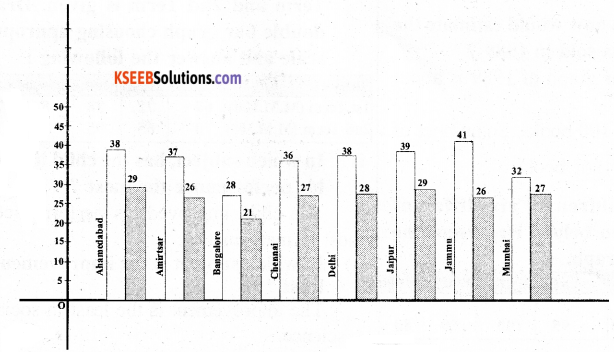i) Which city has the largest difference in the minimum and maximum temperature on the given data ?
Solution:
Given Data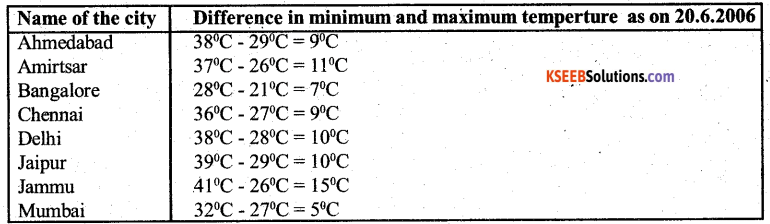Jammu has the largest difference in the minimum and maximum temperature on the given data.

ii) Which is the hottest city and which is the coldest city ?
Solution:
Jammu is the hottest city and Bangalore, is the coldest city.iii) Name two cities where maximum temperature of one was less than the minimum temperature of the other.
Solution:
Bangalore and Jaipur or Bangalore and Ahmedabad.

iv) Name the city which has the least difference between its minimum and, he maximum temperature.
Solution:
Mumbai is the city which has least difference between its minimum and maximum temperature.

error: Content is protected !!